Courses

# Buffer solution - Ionic Equilibrium Chemistry Notes | EduRev

## Physical Chemistry

Created by: Asf Institute

## Chemistry : Buffer solution - Ionic Equilibrium Chemistry Notes | EduRev

The document Buffer solution - Ionic Equilibrium Chemistry Notes | EduRev is a part of the Chemistry Course Physical Chemistry.
All you need of Chemistry at this link: Chemistry

Buffer:

A solution that resists change in pH value upon addition of small amount of strong Acid or Base (less than 1%) is called Buffer solution.

Characteristics of Buffer Solution:

• Constant pH range

• Resist any change

• Addition of small amount of acid, base dilution and temperature

Types of buffer solutions

(A)       Simple Buffer solution

(B)       Mixed Buffer solution

(A)       Simple buffer solution: A solution of weak Acid and weak base in water e.g. CH3COONH4, NH4CN.

Buffer action of simple buffer solution

Consider a simple buffer solution of CH3COONH4, since it is a salt which dissociate completely.

CH3COONH4→ CH3COO + NH4+

If a strong acid such as HCl is added then

HCl→H+ + Cl

The H+ ions from the added acid (HCl) combine with CH3COO ions of form CH3COOHwhich is a weak acid so will not further ionized.

Thus, there is a rise in H+ ion concentration and pH remains constant

CH3COO + H+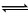CH3COOH (Weak acid)

(B)  Mixed Buffer solutions:

Acidic buffer solution:

An acidic buffer solution consists of solution of a weak acid and its alt with strong base. The best example is mixture of solution of acetic acid and its salt with strong base. (CH3COONa).

CH3COOHCH3COO + H+

C1                        -              -

At equation     C1 – C1α             C1α          C1α

CH3COOH → CH3COO + Na+

C2                     -             -

-                 C2            C2

New equilibrium

CH3COOHCH3COO + H+

C- C1α1               C1α1          C1α1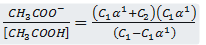C1a1 + C2≈C2

1 - α≈ 1

Kα = C2α1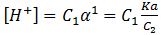pH = -log [H+]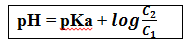This equation is called Handerson equation.

Where C2 = Concentration of common ion

C1 = Concentration of weak acid

Example: Find pH of solution contains 0.1 M (100 ml) CH3COOH and mixed with 0.1 M, 50 ml NaOH. Ka = 105.

Solution: Here, an acid and base is mixed. So, firstly acid – Base Reaction take place.

MIlimoles of CH3COOH = 10 mmol

Milimoles of NaOH = 5 mmol

CH3COOH + NaOH→ CH3COO Na+

10                5

5/150              -                     5/150

Now, solution is having weak acid CH3COOH and it’s salt having common ion. So, this solution is buffer solution Þ Acidic Buffer

pH = pKa + log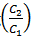pH = pKa + log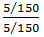pH = 5

Basic Buffer Solution

A basic buffer solution consists of a mixture of a weak base and its salt with strong acid. The best known example is a mixture of NH4OH and NH4Cl.

NH4OHNH4+ OH–                         (weakly ionised)

NH4Cl    → NH4+ + Cl                      (highly ionized)

When a fewe drops of Base (NaOH) are added, the OH ions from NaOH combine with NH4+ to form feebly ionized NH4OH thus there is no rise in the concentration of OH ions and hence the pH value Remains constant.

NH4+ + OH→ NH4OH

pH of basic buffer solution

NH4OHNH4+ + OH

C1                     -           -

C1 – C1aα      C1α      C1α

NH4Cl   →  NH4+ + Cl

C2            -          -

-               C2           C2

At new equilibrium

NH4OHNH4+ + OH

C1 – Cα1          C1α1    C1α1

Kb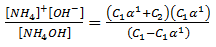1 - α1≈ 1

C1α1 + C≈ C2

K= C2α1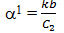[OH] = C1α1 =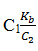Example: 0.1M, 100 ml NH4Cl reacts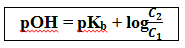with 0.1M, 20 ml Ca(OH)2. Calculate the pH of solution if kb is 105.

Solution :     2 NH4Cl + Ca(OH)2→ 2 NH4OH + CaCl2

Milimoles        0.1 × 100                  0.1 × 20

10                              2

6                                -                  4

Concentration 6                                                   4

120                                            120

Now, solution is having weak base (NH4OH) and its salt NH4Cl which is having ion. Hence this solution acts as buffer

pOH = pKb +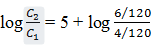pOH = 5.17

pH = 8.83

Example: 0.1 M, 100 ml NH4OH is titrated with 0.1 M, H2SO4 solution. Calculate the pH if kb = 105

2. 25 ml, H2SO4 is added

3. 0.1 M, 20 ml NaOH is mixed in resulting solution for made in part b.

4. Find the pH if 0.1M, 10 ml H2SO4 is added in part b.

Solution

a)         NH4OHNH4+ OH

C1 – C1α       C1α C1α

Kb =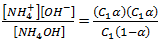After neglection

kb = C1α2

α = 102

pH = 11

b)         When an acid H2SO4 is added with NH4OH (a Base) then acid-Base Reaction takes place.

2 NH4OH + H2SO4→ (NH4)2SO4

milimole               10               2.5                –

5               –                2.5

pOH = 5 + log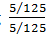pH = 14 – pOH

pH = 9

c)         In part b we are having a weak base and its salt when NaOH is added it will React with (NH4)2SO4.

(NH4)2SO4 + 2 NaOH→ 2 NH4OH + Na2SO4

2.5                  2

Milimoles            1.5                   -                   2

Concentration             1.5/145                -                   2/145

Now, the solution is having weak base and it’s salt, hence Buffer

For (NH4)2SO4→ 2 NH4+ + SO4

Hence, pOH = 5 +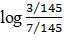pH = 4.632

In part b, when H2SO4 is added it will react with NH4OH.

2NH4OH + H2SO4→ (NH4)2 SO4 + H2O

5                1

3                –                  1

Total milimoles of (NH4)2 SO4 = 7

Total milimoles of NH4OH = 3

pOH = 5 +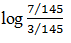pH = 8.63

Buffer Capacity or Buffer Index:

It is the measure of power to resist change in pH of Buffer Solution.

It is defined as the number of moles of acid or Base require by one litre of a Buffer solution for changing its pH by one unit.

Buffer Capacity =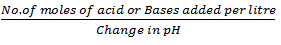Buffer Range:

A solution can act as buffer solution only if ratio of concentration of salt to base or acid is from 1 to 10.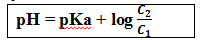pH = pKa + 1

pH = pKa – 1

So, pH range is pKa+ 1

Offer running on EduRev: Apply code STAYHOME200 to get INR 200 off on our premium plan EduRev Infinity!

## Physical Chemistry

77 videos|83 docs|32 tests

,

,

,

,

,

,

,

,

,

,

,

,

,

,

,

,

,

,

,

,

,

;